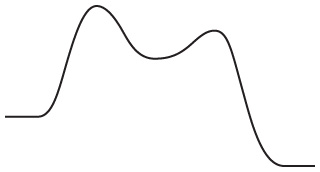# Problem: Consider the following energy diagram showing the energy of a reaction as it progresses:How many elementary steps are involved in this reaction?

⚠️Our tutors found the solution shown to be helpful for the problem you're searching for. We don't have the exact solution yet.

###### Problem Details
Consider the following energy diagram showing the energy of a reaction as it progresses:How many elementary steps are involved in this reaction?